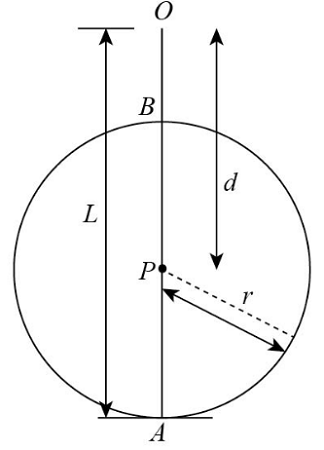# The string in the Figure is L = 135.0 cm long and the distance d to the fixed peg P is 94.5 cm....

## Question:

The string in the Figure is L = 135.0 cm long and the distance d to the fixed peg P is 94.5 cm. When the ball is released from rest in the position shown, it will swing along the dashed arc. How fast will it be going when it reaches the lowest point in its swing? How fast will it be going when it reaches the highest point in its swing?

## Law of Energy Conservation.

The total energy of an isolated body remains constant. If there is not any external force acting on a body, then the sum of kinetic energy and potential energy of the body remains constant.

Given Data

• The length of the string is: {eq}L = 135\;{\rm{cm}} = 1.35\;{\rm{m}} {/eq}.
• The distance of the fixed peg is: {eq}d = 94.5\;{\rm{cm}} {/eq}.The expression to calculate the radius of the circle for ball is,

PA = OA - OP

Substitute the values in the above expression.

{eq}\begin{align*} r &= L - d\\ &= 135\;{\rm{cm}} - 94.5\;{\rm{cm}}\\ &= {\rm{40}}{\rm{.5}}\;{\rm{cm}}\\ &= {\rm{0}}{\rm{.405}}\;{\rm{m}} \end{align*} {/eq}.

There is not any external force on the ball, so the potential energy of the ball will convert into kinetic energy of the ball.

The expression to calculate the potential energy of the ball is,

{eq}P{E_1} = mgL {/eq}.

The expression to calculate the potential energy of the ball is,

{eq}K{E_1} = \dfrac{1}{2}mv_l^2 {/eq}.

Here, {eq}{v_l} {/eq}.is the velocity of the ball at lowest point of the string.

The expression to calculate the velocity of the ball at lowest point of the string is,

{eq}P{E_1} = K{E_1} {/eq}.

Substitute the values in the above expression.

{eq}\begin{align*} mgL &= \dfrac{1}{2}mv_l^2\\ {v_l} &= \sqrt {2gL} \\ &= \sqrt {2\left( {9.8\;{\rm{m}}/{{\rm{s}}^2}} \right)\left( {1.35\;{\rm{m}}} \right)} \\ &= 5.14\;{\rm{m}}/{\rm{s}} \end{align*} {/eq}.

Thus, the velocity of the ball at lowest point of the string is {eq}5.14\;{\rm{m}}/{\rm{s}} {/eq}.

The expression to calculate the distance travelled by the ball from lowest point of string to highest point of string is,

AB = 2r

Substitute the values in the above expression.

{eq}\begin{align*} AB &= 2\left( {{\rm{0}}{\rm{.405}}\;{\rm{m}}} \right)\\ &= 0.81\;{\rm{m}} \end{align*} {/eq}.

The kinetic energy is converted into potential energy at highest point and kinetic energy at highest point.

The expression to calculate the potential energy of the ball at the highest point is,

{eq}P{E_2} = mg\left( {AB} \right) {/eq}.

The expression to calculate the potential energy of the ball at the highest point is,

{eq}K{E_2} = \dfrac{1}{2}mv_h^2 {/eq}.

Here, {eq}{v_h} {/eq}. is the velocity of the ball at highest point of the string.

The expression to calculate the velocity of the ball at highest point of the string is,

{eq}K{E_1} = P{E_2} + K{E_2} {/eq}.

Substitute the values in the above expression.

{eq}\begin{align*} \dfrac{1}{2}mv_l^2 &= mg\left( {AB} \right) + \dfrac{1}{2}mv_h^2\\ v_l^2 &= 2g\left( {AB} \right) + v_h^2\\ {v_h} &= \sqrt {{{\left( {5.14\;{\rm{m}}/{\rm{s}}} \right)}^2} - 2\left( {9.8\;{\rm{m}}/{{\rm{s}}^2}} \right)\left( {0.81\;{\rm{m}}} \right)} \\ &= 3.24\;{\rm{m}}/{\rm{s}} \end{align*} {/eq}.

Thus, the velocity of the ball at lowest point of the string is {eq}3.24\;{\rm{m}}/{\rm{s}} {/eq}.